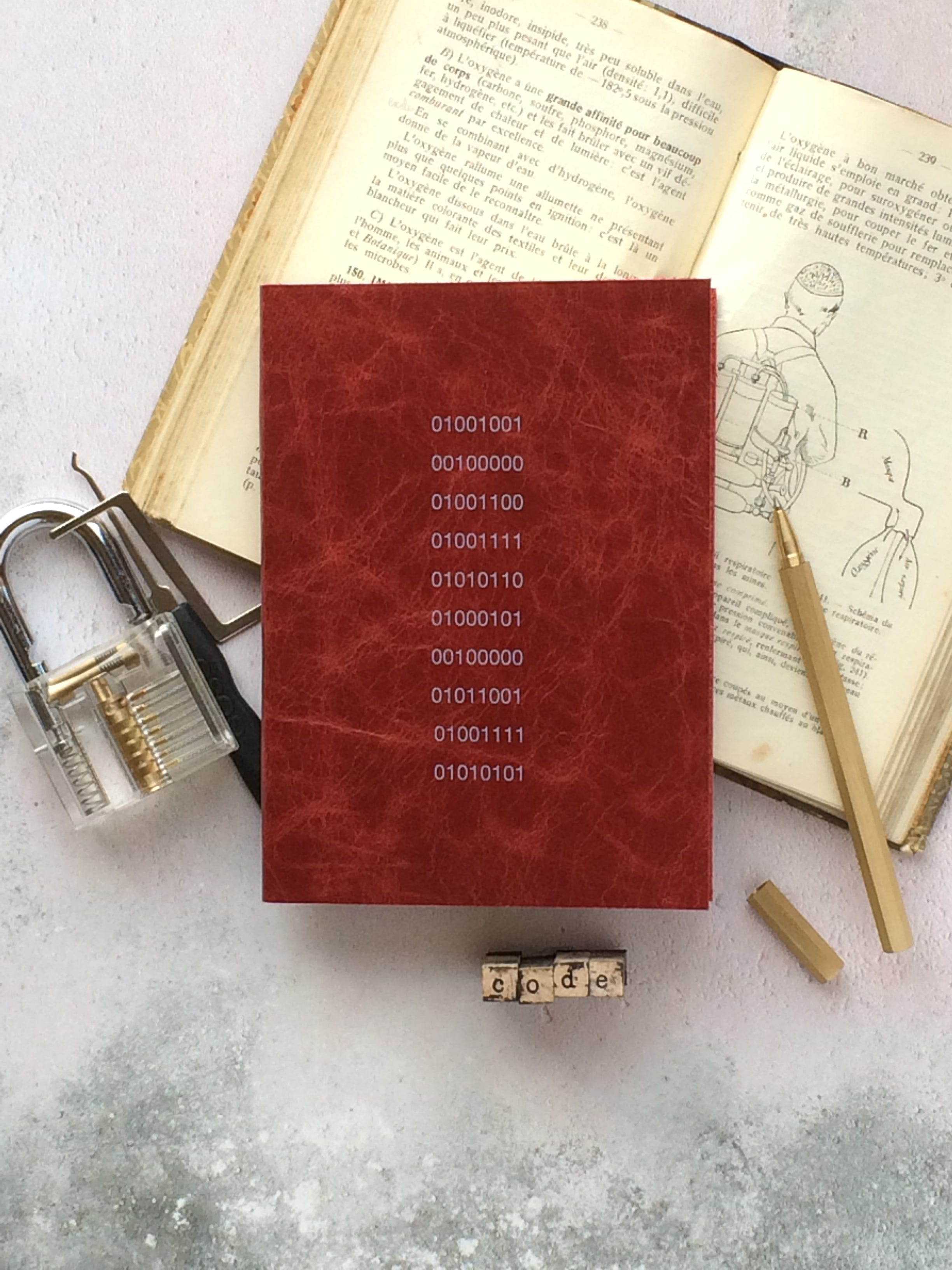# Perceptron: my Neural Net my BFF

Original article was published by Divyosmi Goswami on Artificial Intelligence on Medium# Perceptron: my Neural Net my BFF

## The Perceptron algorithm is the simplest type of artificial neural network. It is a model of a single neuron that can be used for two-class classification problems and provides the foundation for later developing much larger networks.

Introducing Code Me,

It will be a small series that would include code and I will walk through some machine algorithms and implement them in pure python. This is the first blog in the series there will be 7 blogs in total. Actually, my blog let’s classify is a blog of this series but I excluded it. link:- Let’s classify.

So let’s jump into Perceptron:-

What is Perceptron?

In machine learning, the perceptron is an algorithm for supervised learning of binary classifiers. It also helps to make a base class for a multi-class classifier.

In the perceptron, there are only 3 things weights, bias, and input.

The very basic and simple pseudo-code of the perceptron algorithms:-

We at first take our weights as random but that does not work until you are very very lucky to get a goldilocks weight/bias, that is why we need to update the weights hence this equation is used to update it:-

The method of adjusting weights and bias is called Optimization. One of the most important and most used Optimization function is Gradient Descent to be precise Stochastic Gradient Descent.

now as we now about perceptron let’s write some code:-

We will be doing functional programming first and then we will make a class. So we will have the following steps:-

1. Make predict function
2. Train and updating the weights and biases
3. Modeling the data

Data variable for the codes.

Step 1:-

We create a function named predict(x,w), and it will activate the activation function that is:-

`f(x,w) = x index I * w index I +1 + w index I`

Step 2:-

This step is also called a learning loop.

Stochastic gradient descent requires two parameters:

Learning Rate: Used to limit the amount each weight is corrected each time it is updated.
Epochs: The number of times to run through the training data while updating the weight.
These, along with the training data will be the arguments to the function.

There are 3 loops we need to perform in the function:

1. Loop over each epoch.
2. Loop over each row in the training data for an epoch.
3. Loop over each weight and update it for a row in an epoch.

Step 3:-

Now we will need to write a small code to end this topic.

That is the accuracy metric.

And now finally the main.py that is the main code but no the class one.

Step 4:-

Now we will write the class code.

Now I will not write code step by step. I will just write the code.